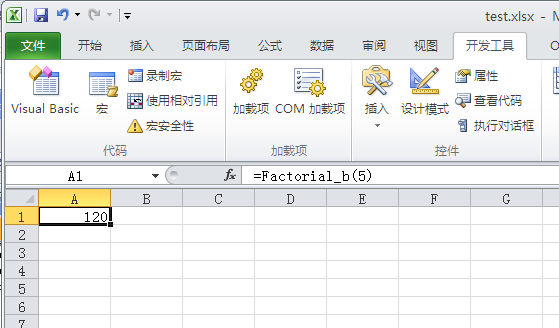• vba代码很简单，如下；数据来源于“国务院办公厅关于2020年部分节假日安排的通知” =workDay(YEAR(TODAY())&"-"&MONTH(TODAY())&"-"&B3) '判断指定日期是否上班 Function workDay(rq) Dim cel As Range If ...
• 在ArcGIS属性表中，一般不会包含实体几何信息，可以采取VBA进行计算。下面是几个简单的代码:1推荐给不会使用AO的朋友;2可以保存为CAL文件以备下次方便使用
• 有网友问到如何求阶层的问题：在Excel中可以直接通过FACT函数来计算非负整数n的阶乘(n!)这里用自定义函数来计算阶乘，使用循环和递归：1.使用循环：FunctionFactorial_a(ByValNumAsInteger)IfNumFactorial_a="#NUM!...

有网友问到如何求阶层的问题：在Excel中可以直接通过FACT函数来计算非负整数n的阶乘(n!)

这里用自定义函数来计算阶乘，使用循环和递归：

1.使用循环：Function Factorial_a(ByVal Num As Integer)

If Num

Factorial_a = "#NUM!"

Else

Factorial_a = 1

For i = 1 To Num

Factorial_a = Factorial_a * i

Next

End If

End Function

2.使用递归：Function Factorial_b(ByVal Num As Integer)

If Num

Factorial_b = "#NUM!"

ElseIf Num = 0 Or Num = 1 Then

Factorial_b = 1

Else

Factorial_b = Num * Factorial_b(Num - 1)

End If

End Function

效果图展开全文• VBA中时间的处理，涉及文本，数字，时间格式，这篇文章用计算两个时间的差值，把这三者使用的函数进行了简述。

VBA中两个时间差值计算：

（1）时间采用文本格式（注：单元格里的时间一般按文本处理）

时间a = TimeValue("11:00:00")
时间b = TimeValue("13:40:00")
时间差 = Abs(DateDiff("n", 时间a, 时间b))

Sub test()
时间a = TimeValue("11:00:00")
时间b = TimeValue("13:40:00")
时间差 = Abs(DateDiff("n", 时间a, 时间b))

Debug.Print 时间差
End Sub

(2)时间采用VBA时间格式

时间a = #11:00:00 AM#
时间b = #1:40:00 PM#
时间差 = Abs(DateDiff("n", 时间a, 时间b))

Sub test2()
时间a = #11:00:00 AM#
时间b = #1:40:00 PM#
时间差 = Abs(DateDiff("n", 时间a, 时间b))

Debug.Print 时间差
End Sub

（3）时间文本数字

文本转时间：

TimeValue("11:00:00")

时间转文本：

Format(#1:40:00 PM#, "hh:mm:ss")

用数字指定时间：

TimeSerial(11, 0, 0)

展开全文excel VBA 时间差
• ' ---------计算程序运行所用时间:---------- Sub GetRunTime() Dim i As Long Dim dteStart As Date Dim strTime As String '关闭屏幕刷新 'Application.ScreenUpdating = False dteStart = Timer '----...

' ---------计算程序运行所用时间:----------
Sub GetRunTime()
Dim i As Long
Dim dteStart As Date
Dim strTime As String

'关闭屏幕刷新
'Application.ScreenUpdating = False
dteStart = Timer

'---------运行过程主体-------

strTime = Format((Timer - dteStart), "0.00000")

Msgbox "运行过程: " & strTime & "秒"

‘打开屏幕刷新
'Application.ScreenUpdating = True

End Sub

展开全文vba
• Sub TEST() Dim i As Integer Range("d1:d255").Cut Range("b1:b255") Range("e1:e255").Cut Range("d1:d255") For i = 1 To 255 cells(6,i*3)="= round(average(range(b"&(i*3-2)&":b"&...

Sub 另存本表为TXT文件()

Dim i As Integer
Range("d1:d255").Cut Range("b1:b255")
Range("e1:e255").Cut Range("d1:d255")
For i = 1 To 125
If Cells(i * 3, 1) <> "" Then
Cells(i * 3, 6) = UCase(Left(Cells(i * 3, 1), Len(Cells(i * 3, 1)) - 2))
Cells(i * 3, 7) = Round((Cells(i * 3 - 2, 2) + Cells(i * 3 - 1, 2) + Cells(i * 3, 2)) / 3, 3)
Cells(i * 3, 8) = Round((Cells(i * 3 - 2, 3) + Cells(i * 3 - 1, 3) + Cells(i * 3, 3)) / 3, 3)
Cells(i * 3, 9) = Round((Cells(i * 3 - 2, 4) + Cells(i * 3 - 1, 4) + Cells(i * 3, 4)) / 3, 3)
Sheet2.Range("a1:d100").Value = Sheet1.Range("f1:i100").Value
End If
Next

Dim j As Long

For j = Sheet2.Cells(1048576, 2).End(xlUp).Row To 1 Step -1

If Sheet2.Cells(j, 1) = "" Then           '如果单元格为空白

Sheet2.Cells(j, 1).EntireRow.Delete          '符合条件的都删除整行

End If

Next                             '检测下一个

Dim s As String

Dim FullName As String, rng As Range

Application.ScreenUpdating = False

FullName = (ActiveSheet.Name & ".txt")   '以当前表名为TXT文件名

'   FullName = Replace(ThisWorkbook.FullName, ".xls", ".txt")  '以当前文件名为TXT文件名

'   FullName = Replace(ThisWorkbook.FullName, ".xls", ActiveSheet.Name & ".txt")  '以文件名&表名为TXT文件名

Open FullName For Output As #1    '以读写方式打开文件，每次写内容都会覆盖原先的内容

'参考帮助，fullname为文件全名

For Each rng In Sheet2.Range("a1").CurrentRegion

s = s & IIf(s = "", "", ",") & rng.Value

If rng.Column = Sheet2.Range("a1").CurrentRegion.Columns.Count Then

Print #1, s & " "   '把数据写到文本文件里

s = ""
'          Else
'
'          Print #1, s & ""   '把数据写到文本文件里
'
'            s = ""
End If

Next

Close #1    '关闭文件

Application.ScreenUpdating = True

MsgBox "数据已导入文本"
End Sub

展开全文python
• Len 函数 返回 Long，其中包含字符串内字符的数目，或是存储一变量所需的字节数。 语法 Len(string | varname) Len 函数的语法有下面这些部分： 部分 ...如果 varname 包含 Null，会返回 N...
• 如何用VBA计算个人所得税

千次阅读 2018-06-09 16:47:23
Sub i个人所得税()For Each Sheet In WorksheetsFor i = 2 To xx = Sheet.UsedRange.Rows.CountDim a%, b% a = Sheet.Cells(i, "I") If a &lt; 3500 Then Range(Sheet.Cells(i, "...
• VBA计算数独表格自动运算,所有符合数独表格输出
• Option Explicit Sub main_fact() Dim n As Long Dim i As Integer, b As Double, sum As Double sum = 0 n = InputBox("请输入N") For i = 1 To n b = fact(i) sum = sum + b Next ...Public Func...Excel VBA
• 作为一名执著的 C# 程序员，当一位学经济的老友从国外找来，让我帮他写完 VBA 作业的时候，一开始，其实我是拒绝的。 各路VB大神，不要嘲笑在下啊，我可是足足用了一个小时才在 Excel 里到了编写 VB 代码的地方 ...
• 通用工资计算(VBA excel),各种任意工资列项，都可以使用。随意设定，计算简便，打印功能。
• 计算两个日期之间的工作日，用VBA，因量大，最好用数组做 Sub kk() Dim arr, i&amp;, j&amp;, m&amp; arr = Sheet2.Range("b3:f4") For i = 1 To UBound(arr) m = 0...
• 是精确计算圆周长、圆面积、球体积等几何形状的关键值。 在分析学里，π可以严格地定义为满足sin x = 0的最小正实数x。圆周率用希腊字母 π表示，是一个常数（约等于3.141592654），是代表圆周长和直径的比值。它是...
• 对于Excel来说几乎是家喻户晓，但人们对Excel-VBA了解并不普遍。VBA是Visual Basic for Application的缩写，是集成在Office产品中的开发语言和开发环境。掌握VBA可以使办公效率变得更快。特别在统计分析方面，VBA...
• 湿球温度迭代换算，EXCEL制作，基于迭代换算，精度高，由温度，湿度，气压三个数据计算，使用时请启用宏
• PPT+VBA实现计时（倒计时）展示，可用于高考倒计时或者系统运行时间计时展示，不用编程。PPT+VBA实现计时（倒计时）展示，可用于高考倒计时或者系统运行时间计时展示，不用编程ppt vba
• 1、 编写程序，随机生成20个两位整数，并统计出其中小于等于60、大于60小于等于80及大于80的数据以及相应个数，结果打印输出到窗体。 Private Sub Form_Click() For i = 1 To 20 a = Fix(Rnd() * 91 + 10) ...
• VBA具有强大的计算功能。除常规四则运算以外，还能够进行文本连接、乘幂运算、比较运算等。 文本连接 在VBA中，允许将多个文本通过文本连接符(&)进行连接并组合成新的文本，其基本语法为： Exp1 & Exp2 ...
• 计算机二级--VBA程序 考试知识点全面归纳8.3.3 数据类型和数据库对象Access数据库系统创建表对象时所涉及的字段数据类型(除了OLE对象和备注数据类型外)，在VBA中都有数据类型相对应。 一、标准数据类型传统的BASIC...
• Excel_VBA计算数组的长度

千次阅读 2012-09-29 15:09:15
UBound 函数 返回一个 Long 型数据，其值为指定的数组维可用的最大下标。 LBound 函数 返回一个 Long 型数据，其值为指定数组维可用的最小下标。...计算数组的长度： length = UBound(a) - LBound(a) + 1 ...
• VBA小程序_获取单元格里面出现多少个字符.xlsm
• 在长时间运算中使用友好提示_Excel VBA应用.rar,如果某段VBA代码需要较长的执行时间，为了不让使用者在不明就里的状态下等待程序运行，甚至认为Excel死机，VBA开发人员应该使用友好的提示，用户告知使用者当前的状况...Excel应用
•EXCEL管理系统
• 使用excel工具、利用BS模型求合理的期权定价。...虽然BS公式有analytic form，但是隐含波动率并不存在一个closed-form Solution，实际中常用数值方法来计算implied 波动率。最常用的是Newton-Raphson迭代方法。
• 高程点批量处理、方格网、断面图土方计算、等高线作图
• 满意答案l7671112352018.07.03采纳率：58%等级：12已帮助：5250人可以.如下Public Sub GetMyIP()Dim strComputer As StringDim objWMI As ObjectDim colIP As ObjectDim IP As ObjectDim i As IntegerstrComputer = ...
• Sub 查询数据()Dim tt = TimerRange("I2")=Application.WorksheetFunction.VLookup(Range("H2"), Range("A:F"), 2, False)Range("J2")=Application.WorksheetFunction.VLookup(Range("H2"), Range("A:F"), 3, False)......